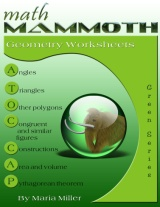You are here: HomeOnline resourcesGeometry → The Pythagorean theorem

# The Pythagorean Theorem: online activities, tutorials, and worksheets

This is a hand-picked list of online activities, tutorials, and worksheets concerning the The Pythagorean Theorem.

## Pythagorean Theorem

Pythagorean Theorem
Investigate the areas of the squares on the sides of right angled triangles using this interactive figure.
www.interactive-maths.com/pythagoras-theorem-ggb.html

Proof Without Words: Pythagorean Theorem
Watch a dynamic, geometric "proof without words" of the Pythagorean Theorem. Can you explain the proof?
illuminations.nctm.org/Activity.aspx?id=4211

Pythagorean Explorer
Practice calculating the missing side length of right triangles. Includes three difficulty levels.
www.shodor.org/interactivate/activities/PythagoreanExplorer

The Pythagorean Theorem - a session from Geometry course by Learning Math
An online course that includes tutorials, problems, videos, interactive activities, homework problems and solutions. In this session, you will look at a few proofs and several applications of one of the most famous theorems in mathematics: the Pythagorean theorem.
www.learner.org/courses/learningmath/geometry/session6/index.html

A virtual manipulative: The Pythagorean Theorem
Solve two puzzles that illustrate the proof of the Pythagorean Theorem.
nlvm.usu.edu/en/nav/frames_asid_164_g_3_t_3.html

Pythagorean Theorem Lesson
This lesson introduces and explores the Pythagorean Theorem using the Pythagorean Explorer. Three computer activities give students the opportunity to observe triangles, learn and use the Pythagorean Theorem and practice different ways of determining areas of triangles.
www.shodor.org/interactivate/lessons/pyth.html

Pythagorean Theorem for elementary school?
This is a lesson plan showing how you could introduce the Pythagorean Theorem in elementary school. I don't really recommend such because I feel it belongs to the middle school, but it seems some student-teachers are asked to write a lesson for such.
/teaching/pythagorean-theorem-elementary.php

## WorksheetsMath Mammoth Geometry Worksheets Collection
A collection of quality worksheets with variable problems for grades 3-8. Topics include angle relationships, triangles, quadrilaterals, congruency, similar figures, constructions, area, volume, and the Pythagorean Theorem. Price: \$9.00 download. See the free samples!
www.mathmammoth.com/worksheets/geometry.php

Maisonet Math
Free PDF worksheets where you can practice finding the unknown length of the hypotenuse of a right triangle or find the length of a missing leg!
www.mrmaisonet.com/index.php?/Pythagorean-Theorem/View-category.html

Let's Practice Geometry - Free Worksheets
Lots of free worksheets for high school geometry, including topics such as the Pythagorean Theorem, perimeter, area, volume, angle relationships, triangle theorems, similarity and congruence, logic, proofs, trig, polygons, and circles.
www.letspracticegeometry.com/free-geometry-worksheets

Geometry worksheets at AGMath.com
Hundreds of free PDF worksheets for high school geometry topics, including geometric constructions, triangle congruence, circle, area, the Pythagorean Theorem, solid geometry, and similarity. The worksheets are loosely based on the "Discovering Geometry" textbook by Michael Serra. Compiled by math teacher Jason Batterson.
AGmath.com/1973.html

## Books

Geometry: A Guided InquiryAn excellent choice for high school geometry. I've written an in-depth review of this book and of its supplement Home Study Companion - Geometry, latter by David Chandler.

Harold Jacobs GeometryA popular choice for geometry among homeschoolers, and well written. Please read my review.

Dr. Math Presents More Geometry
An inexpensive companion to any high school geometry course with excellent explanations. Read my review.

#### Key to Geometry Workbooks

Here is a non-intimidating way to prepare students for formal geometry. Key to Geometry workbooks introduce students to a wide range of geometric discoveries as they do step-by-step constructions. Using only a pencil, compass, and straightedge, students begin by drawing lines, bisecting angles, and reproducing segments. Later they do sophisticated constructions involving over a dozen steps-and are prompted to form their own generalizations. When they finish, students will have been introduced to 134 geometric terms and will be ready to tackle formal proofs.